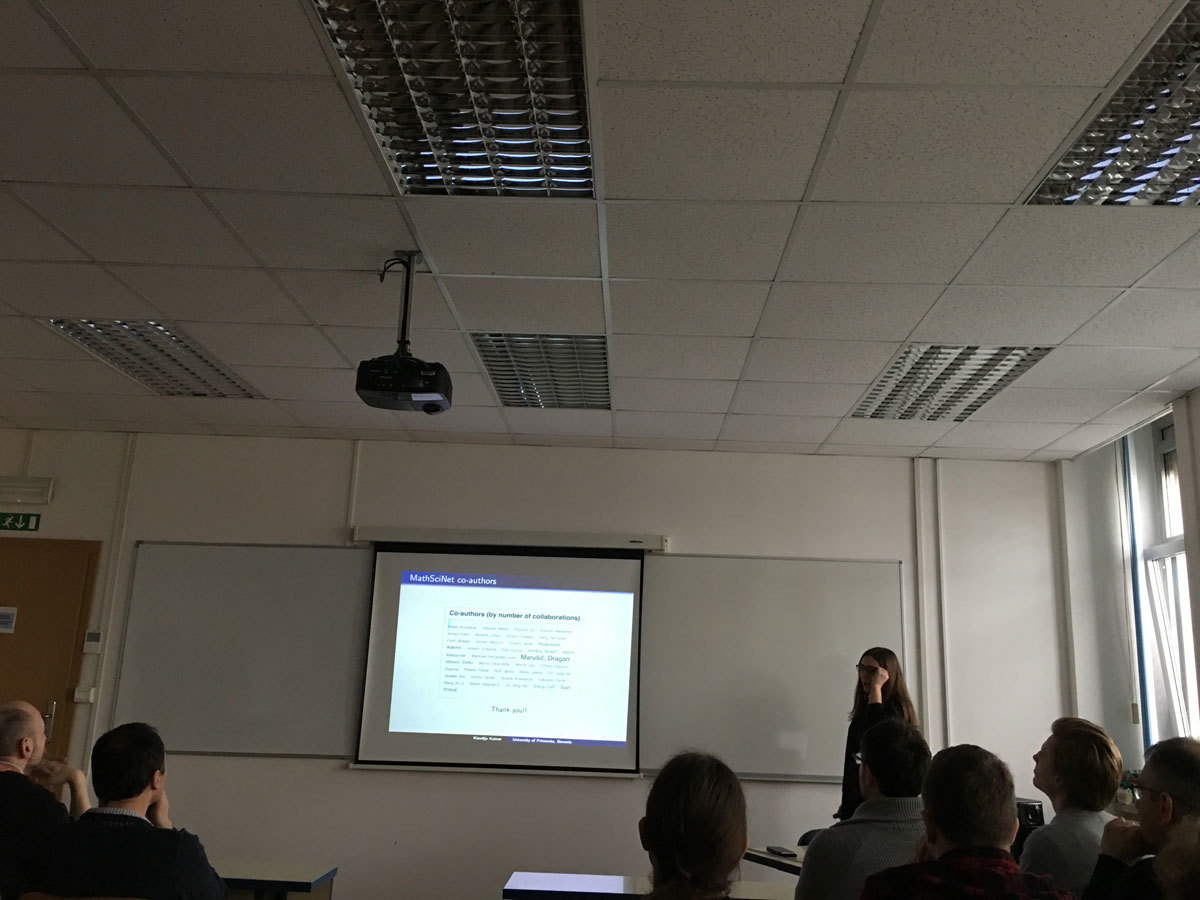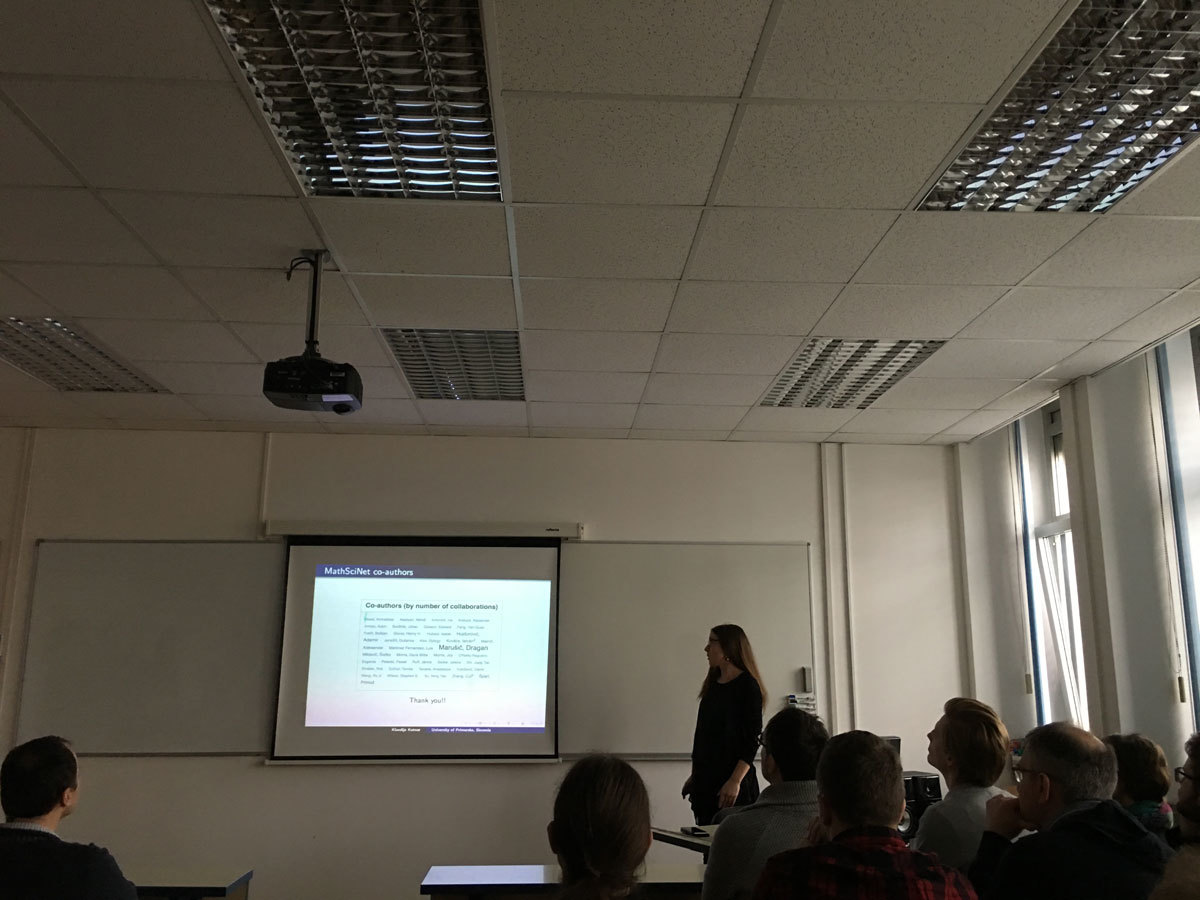Univerza na Primorskem Fakulteta za matematiko, naravoslovje in informacijske tehnologije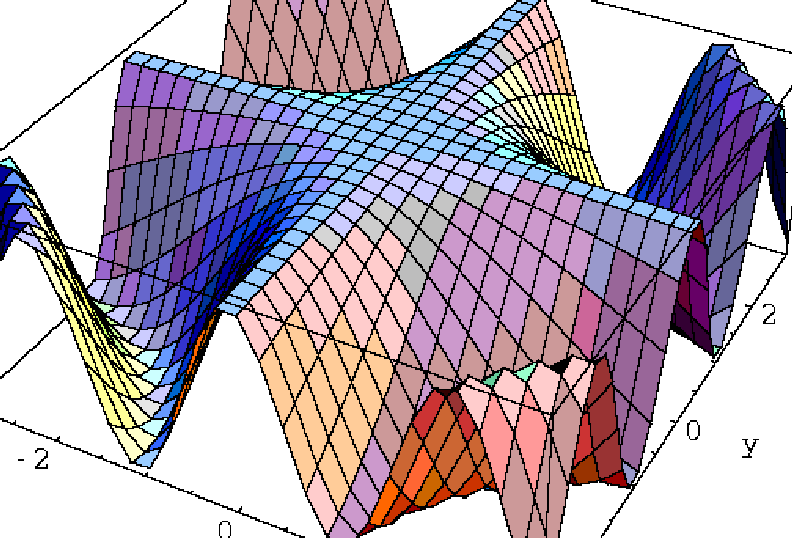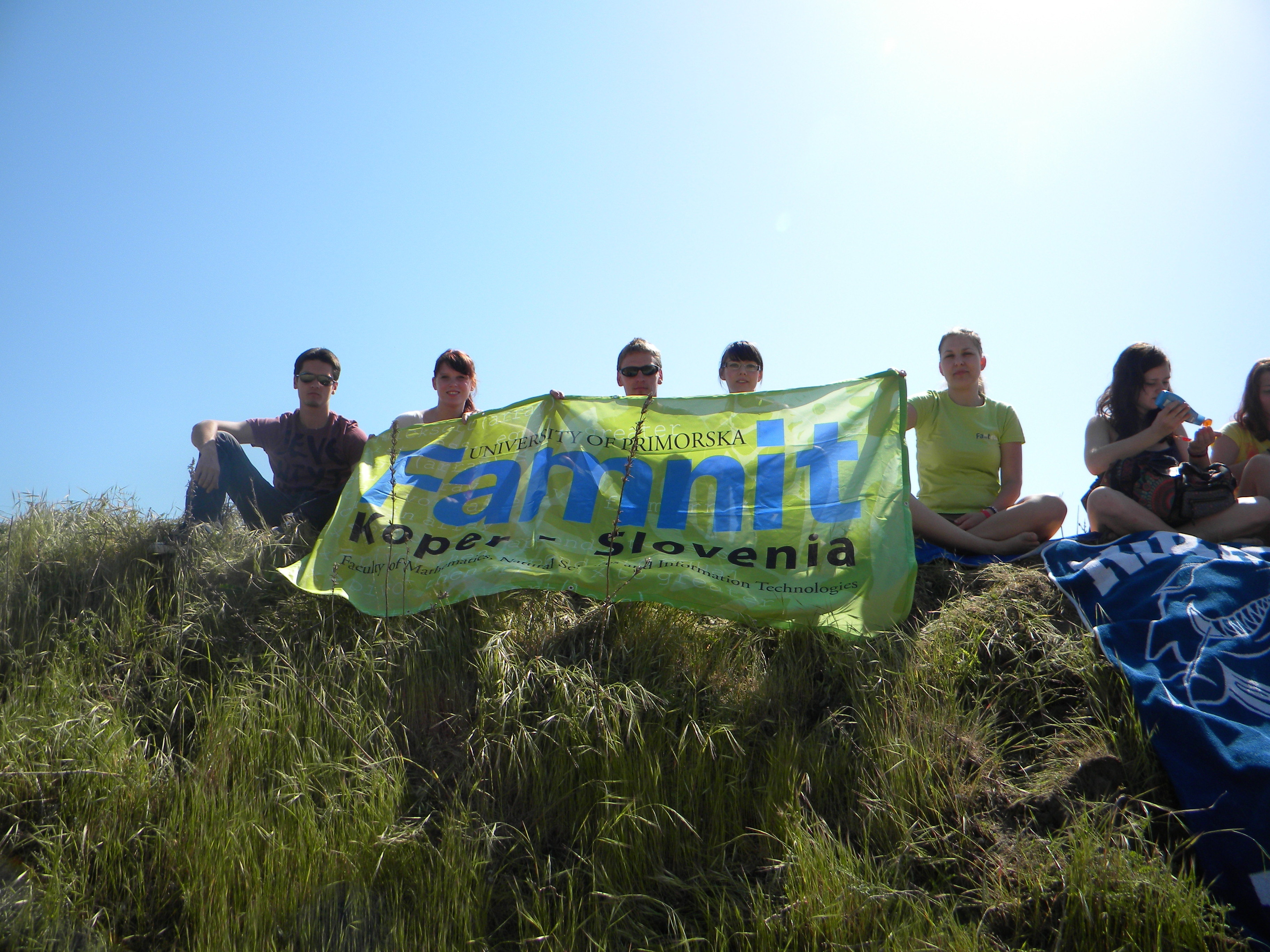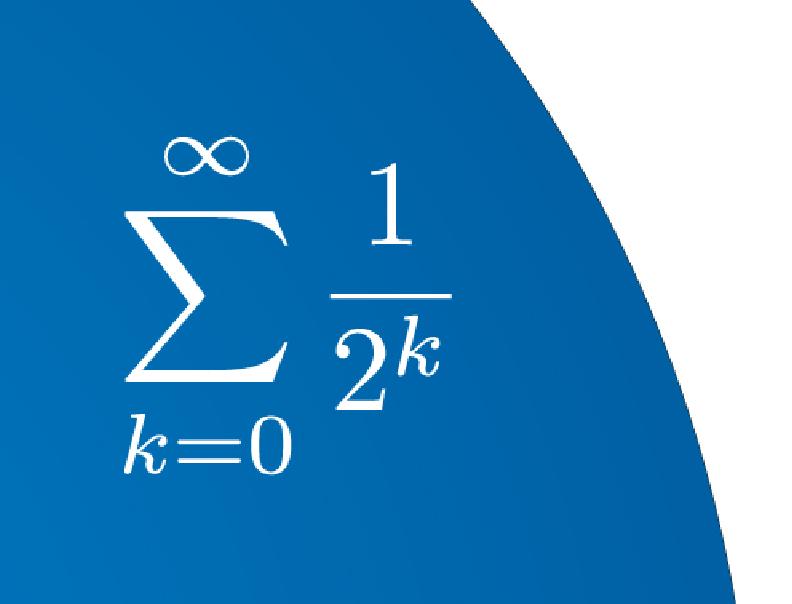SI | EN# Raziskovalni matematični seminar - Arhiv

 2021 2020 2019 2018 2017 2016 2015 2014 2013 2012 2011 2010
 1 2 3 4 5 6 7 8 9 10 11 12
Datum in ura / Date and time: 27.3.18
(10:00-11:00)
Predavalnica / Location: FAMNIT-VP
Predavatelj / Lecturer: Enes Pasalic
Naslov / Title: Discrete mathematical structures suitable for applications in cryptography
Vsebina / Abstract:

In this talk I will give a brief survey of my research in the field of cryptography conducted for the past twenty years. The importance of developing discrete mathematical structure suitable for real-life applications in symmetric-key encryption schemes (and cryptography in general) will be motivated on a non-professional level (students will not be bored), thus rather providing a big picture than insisting on unnecessary details related to my scientific work. Some highlights of my research work will be mentioned related to both applicative aspects of discrete mathematical structures, such as the use of Boolean functions to design orthogonal sequences for CDMA applications, and also to purely mathematical context such as the construction of semi-fields and projective/affine spaces. This will lead us to some nice problems (open for more than 20 years) that concern the theory of finite fields or Boolean functions which can be formulated in a compact and elegant way. These problems are exactly the kind of problems that arise our intrigue and give us a motivation to keep on with research. The issue of academic freedom to deal with these »hard« problems will be also briefly addressed.

Datum in ura / Date and time: 26.3.18
(10:00-11:00)
Predavalnica / Location: FAMNIT-POŠTA
Predavatelj / Lecturer: Matjaz Kovse (Indian Institute of Technology Bhubaneswar)
Naslov / Title: Distance matrices of partial cubes
Vsebina / Abstract:

Partial cubes are isometric subgraphs of hypercubes. Median graph is a graph in which every three vertices u,v and w have a unique median: a vertex m that belongs to shortest paths between each pair of u,v and w. Median graphs present one of the most studied subclasses of partial cubes. We determine the Smith normal form of the distance matrices of partial cubes and the factorisation of Varchenko determinant of product distance matrices of median graphs.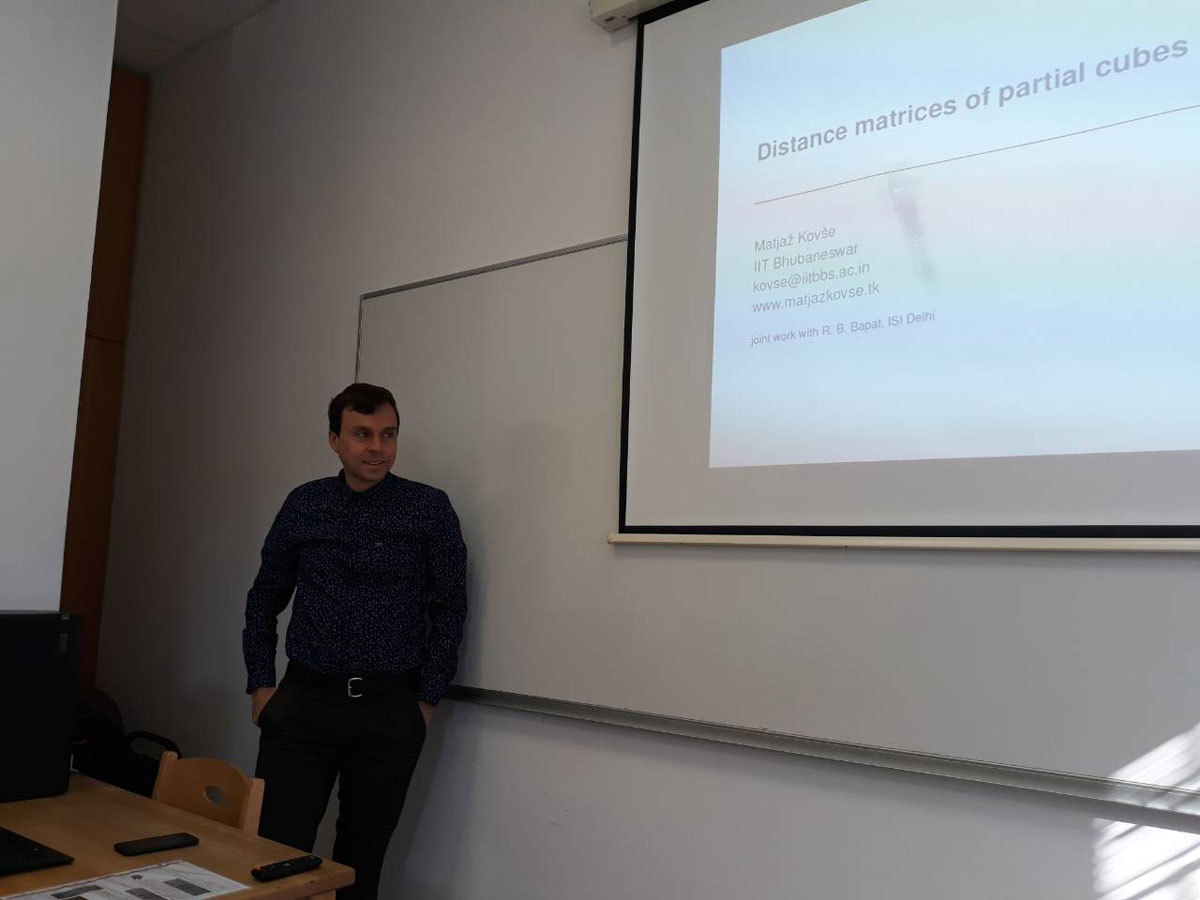Datum in ura / Date and time: 27.3.18
(11:00-12:00)
Predavalnica / Location: FAMNIT-POŠTA
Predavatelj / Lecturer: Joszef Lörinczi (Loughborough University, UK)
Naslov / Title: Non-local operators and jump processes
Vsebina / Abstract:

The deep relationship between the Laplacian, heat equation and Brownian motion has been explored with much success over the past decades. Recently much attention has been attracted by fractional Laplacians, non-local equations and jump processes allowing to capture new phenomena and offer a new prespective on the classical theory. I will highlight some aspects of a probabilistic understanding of solutions of non-local equations involving some specific models.

Datum in ura / Date and time: 16.3.18
(10:00-11:00)
Predavalnica / Location: FAMNIT-VP
Predavatelj / Lecturer: Cheryl E. Praeger (University of Western Australia)
Naslov / Title: Breaking down barriers in Mathematics
Vsebina / Abstract:

Mathematics is all around us. It’s behind all the algorithms that make computers work so anything that involves computing and technology needs maths. If you can do maths you are essentially able to invent the future.

When I was a student I was told “there are no jobs for a maths graduate”. Clearly wrong. I’ll tell you a bit of my journey into maths, the kinds of maths I love and use - the mathematics of symmetry - my links with mathematics in Slovenia - how this is a link between mathematics and life - how there is a place in maths for both women and men.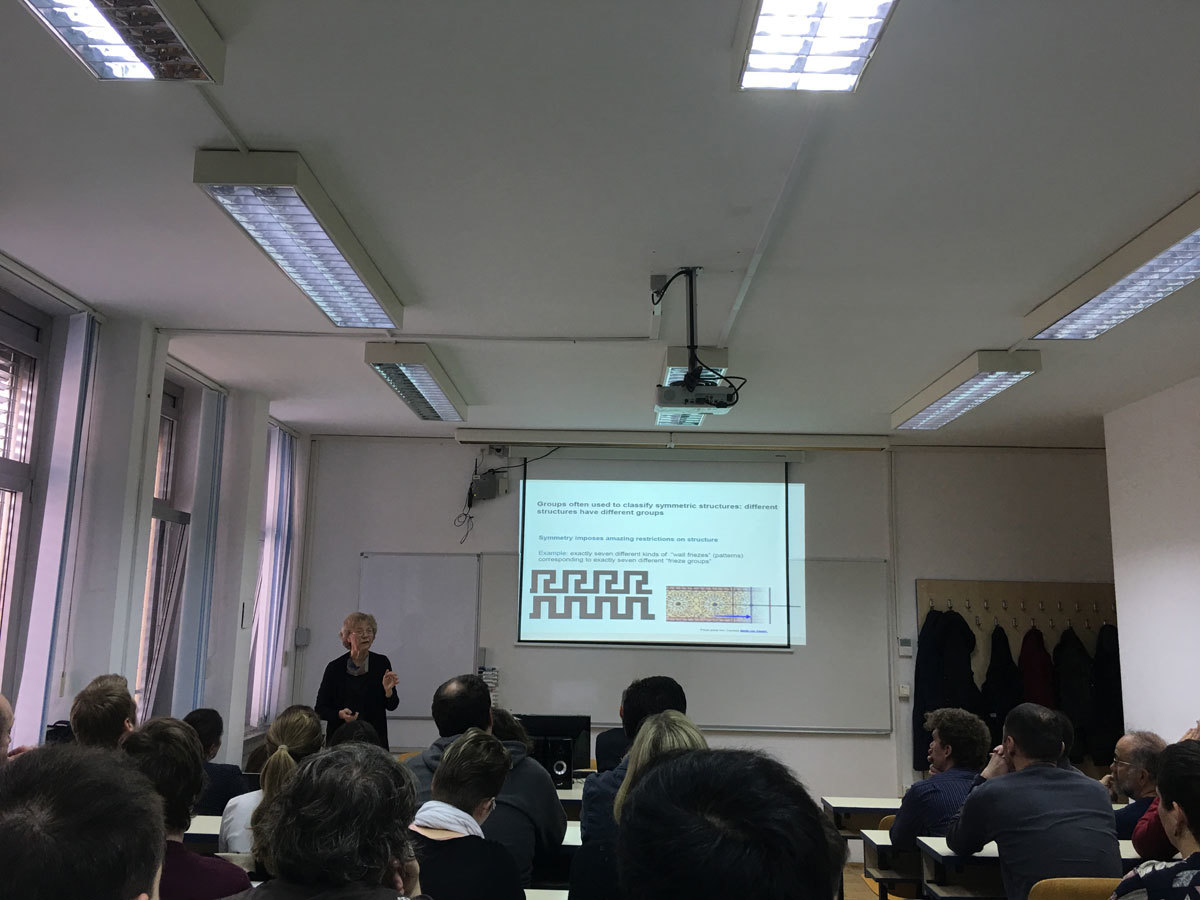Datum in ura / Date and time: 19.3.18
(10:00-11:00)
Predavalnica / Location: FAMNIT-POŠTA
Predavatelj / Lecturer: Slobodan Filipovski (UP IAM & UP FAMNIT)
Naslov / Title: On extremal graphs of given degree and diameter/girth
Vsebina / Abstract:

In this talk we present the main topics, research questions and the expected results of the proposed PhD thesis.

In the PhD Thesis we will focus on open questions and problems which concern the Cage problem and the Degree/Diameter problem for undirected and directed graphs.

Cage Problem (Degree/Girth Problem): Given natural numbers k and g, find the smallest possible number of vertices n(k,g) in a graph of degree k and girth g.

Degree/Diameter Problem: Given natural numbers k and d, find the largest possible number of vertices n(k,d) in a graph of maximum degree k and diameter d.

(Directed) Degree/Diameter Problem: Given natural numbers d and k, find the largest possible number of vertices n_{d,k} in a digraph of maximum out-degree d and diameter k.

In the first part of the PhD thesis we will focus on the bipartite graphs of excess 4, antipodal cages of even girth and small excess and on the excess of the vertex-transitive graphs. We plan to prove the non-existence of infinitely many bipartite (k,g)-graphs of excess 4, the non-existence of almost all potential antipodal cages of even girth and small excess and to improve the Biggs's result which address the existence of the vertex-transitive graphs of given degree and girth.

In the second part we will focus on the Bermond and Bollob\' as question: Given a positive integer c>0, does there exist a pair k and d, such that n(k,d)\leq M(k,d)-c?,'' where M(k,d) is the Moore bound. We plan to answer this question combining spectral and real analysis.

In the last part of the PhD thesis we will focus on two problems which concern the degree/diameter problem for directed graphs. We plan to answer the question about the monotonicity of the function n_{d,k} in k and d, and to prove the non-existence of infinitely many (d,k,\delta)-digraphs containing only self repeat vertices.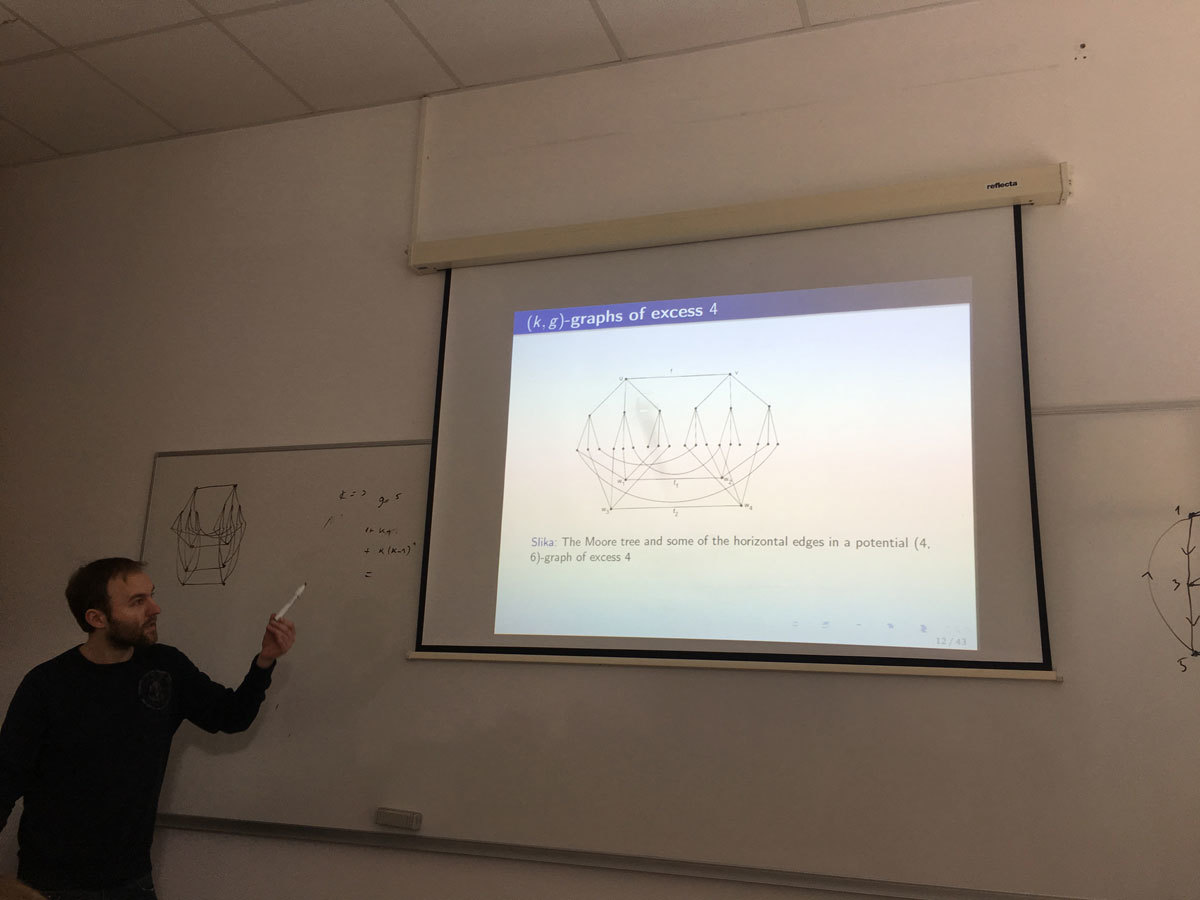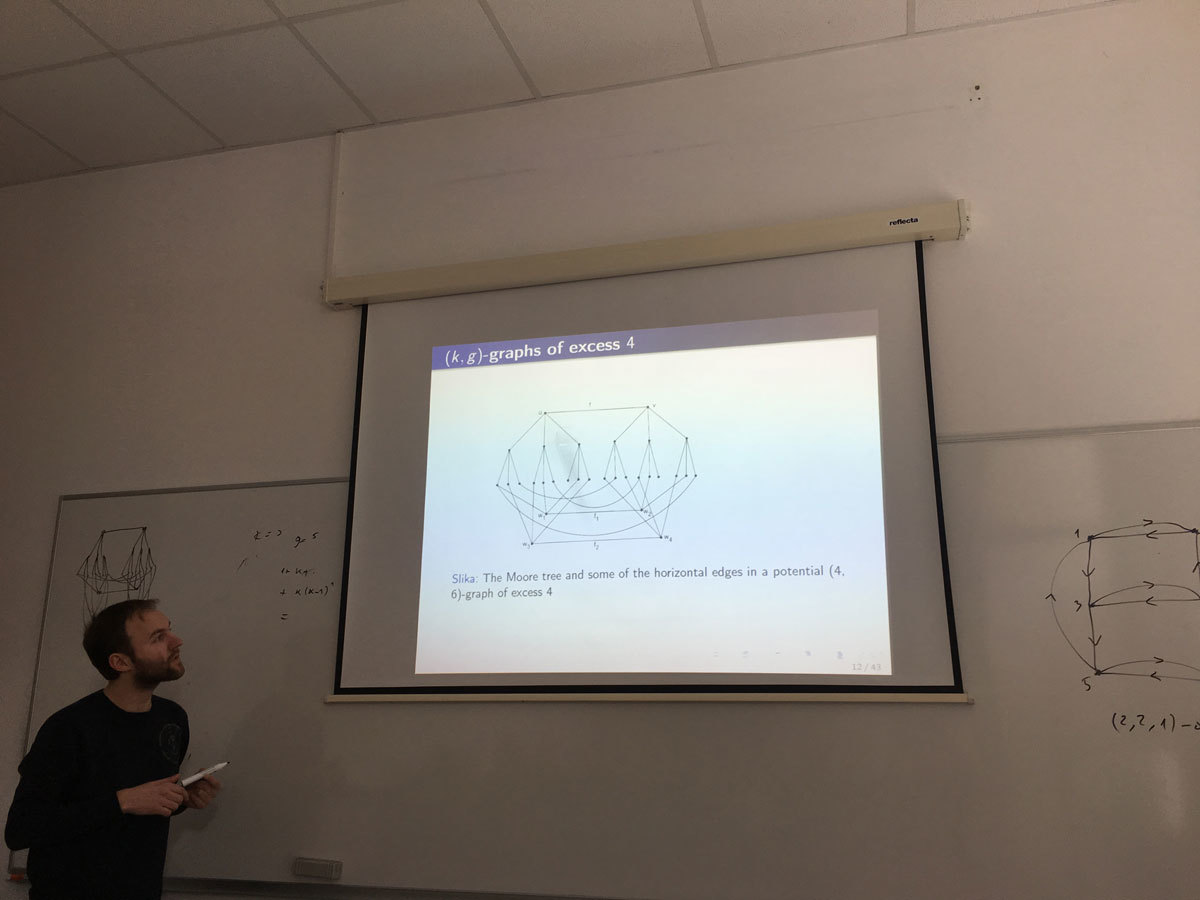Datum in ura / Date and time: 12.3.18
(10:00-11:00)
Predavalnica / Location: FAMNIT-POŠTA
Predavatelj / Lecturer: Emanuele Perazzolo (University of Verona, Italy)
Naslov / Title: (n + 1)-coloured graphs: a combinatorial representation for PL n-manifolds
Vsebina / Abstract:

This thesis would like to extend some concepts of an existing representation theory for closed PL manifolds, called Crystallization Theory. This theory has the peculiarity of using a particular class of regular edge-coloured (multi)graphs (called crystallizations) to represent PL manifolds, so that topological properties can be translated into combinatorial ones (PL invariants of manifolds can be computed directly on the representing graphs).

Firstly, we introduce concepts on Combinatorial Topology and on Graph Theory in order to construct such particular graphs. Then, properties, classic results and the computation of an important topological invariant (Fundamental Group) will be mentioned.

In the main part of the work we study a local operation on a graph, called switching of a \rho-pair, which allows to minimize a graph without changing the underlying PL structure and generalize its property to graphs representing general polyhedra (a sketch of the proof is given).

Then, we focus on the 3-dimensional case. After some basic definitions, we prove that the relations between Heegaard splittings or diagrams and edge-coloured graphs still hold for manifolds with non-empty boundary and that the classical topological invariant, the Heegaard genus, and the totally combinatorial invariant, the regular genus, coincide for all compact 3-manifolds (again a sketch of the proof is described).

Finally, we study the connections between planarity of graphs and regular planar embeddings of edge-coloured graphs: we establish a little result concerning non-planarity of simple graphs that allows to exclude planar simple graphs as representatives of PL n-manifolds, with n\ge 3.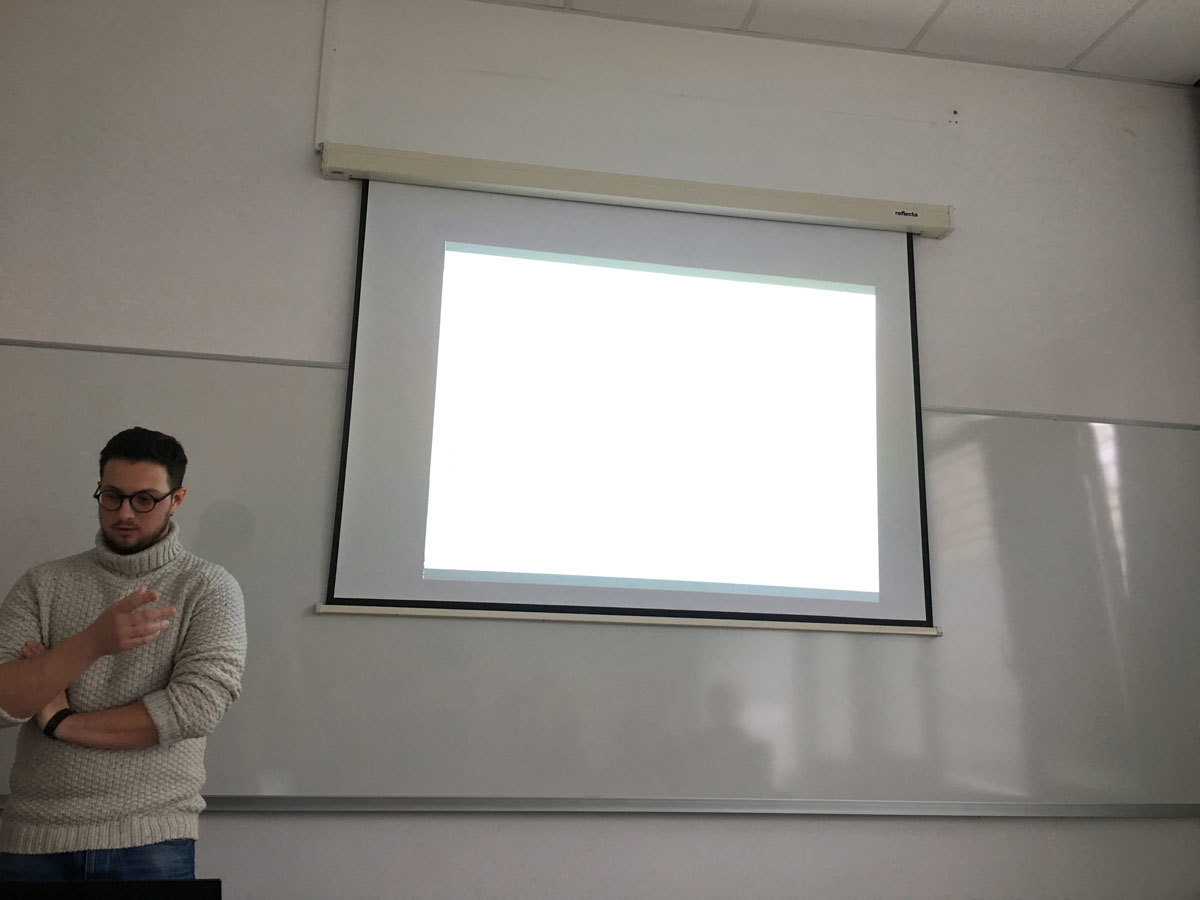Datum in ura / Date and time: 12.3.18
(11:00-12:00)
Predavalnica / Location: FAMNIT-POŠTA
Predavatelj / Lecturer: Nastja Cepak (UP IAM & UP FAMNIT)
Naslov / Title: On bent functions lying outside the completed Maiorana-McFarland class and permutations via translators
Vsebina / Abstract:

One of the most well known classes of bent functions is the Maiorana-McFarland class M. Knowing in which ways it overlaps with other classes of bent functions and determining whether new constructions yield bent functions that lie outside or inside of it are important questions. We will take a look at a special version of the Rothaus'construction and see if it can generate bent functions lying outside the completed Maiorana-McFarland class M#. Sufficient conditions will be presented for functions within C (and D) class to lie outside the M# class and we will demonstrate examples of such functions in C and show two infinite classes of functions in D outside the M# class. Next, translators will be considered - first linear translators and then their generalisation, the Frobenius translator. Constructions of permutations and bent functions relying on translators will be demonstrated. Finally, constructions of vectorial plateaued functions, permutations and complete permutations using the Maiorana-McFarland class.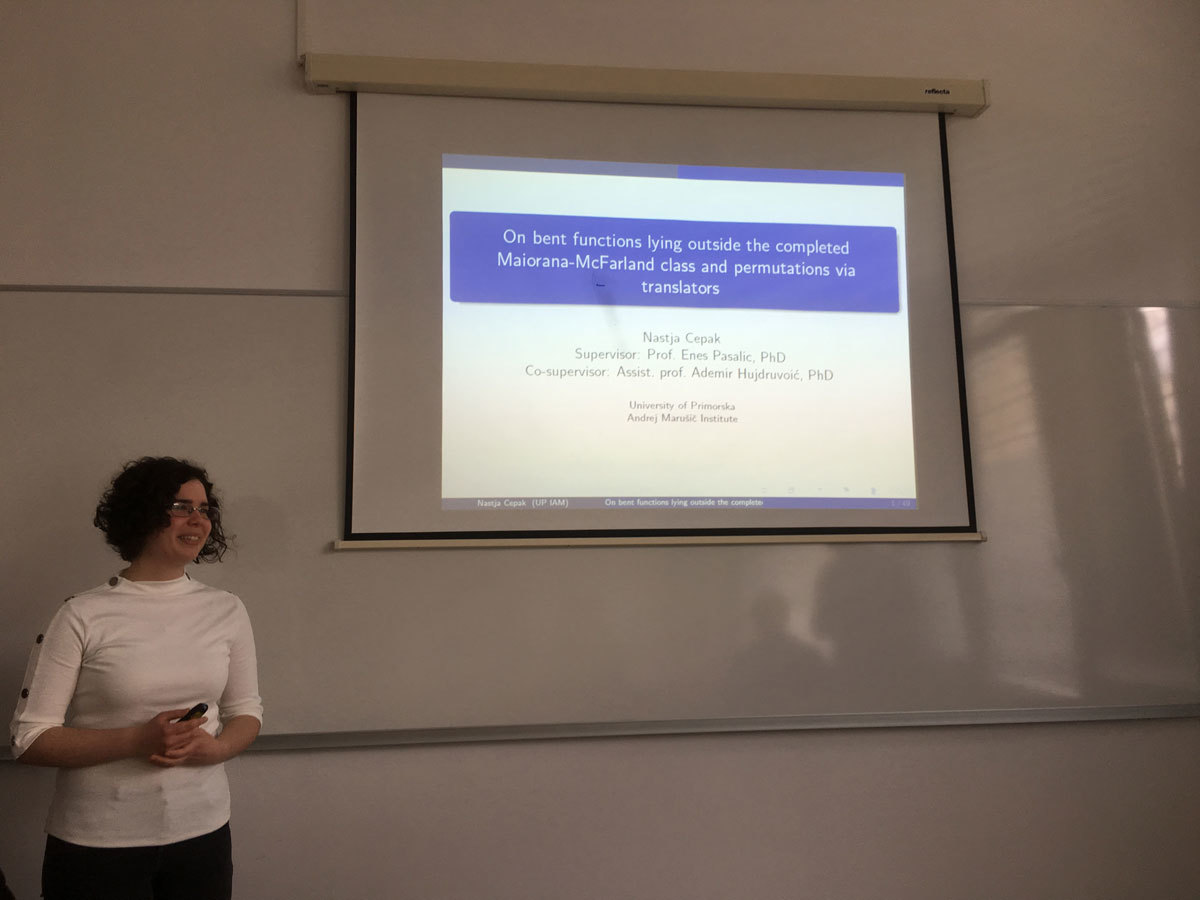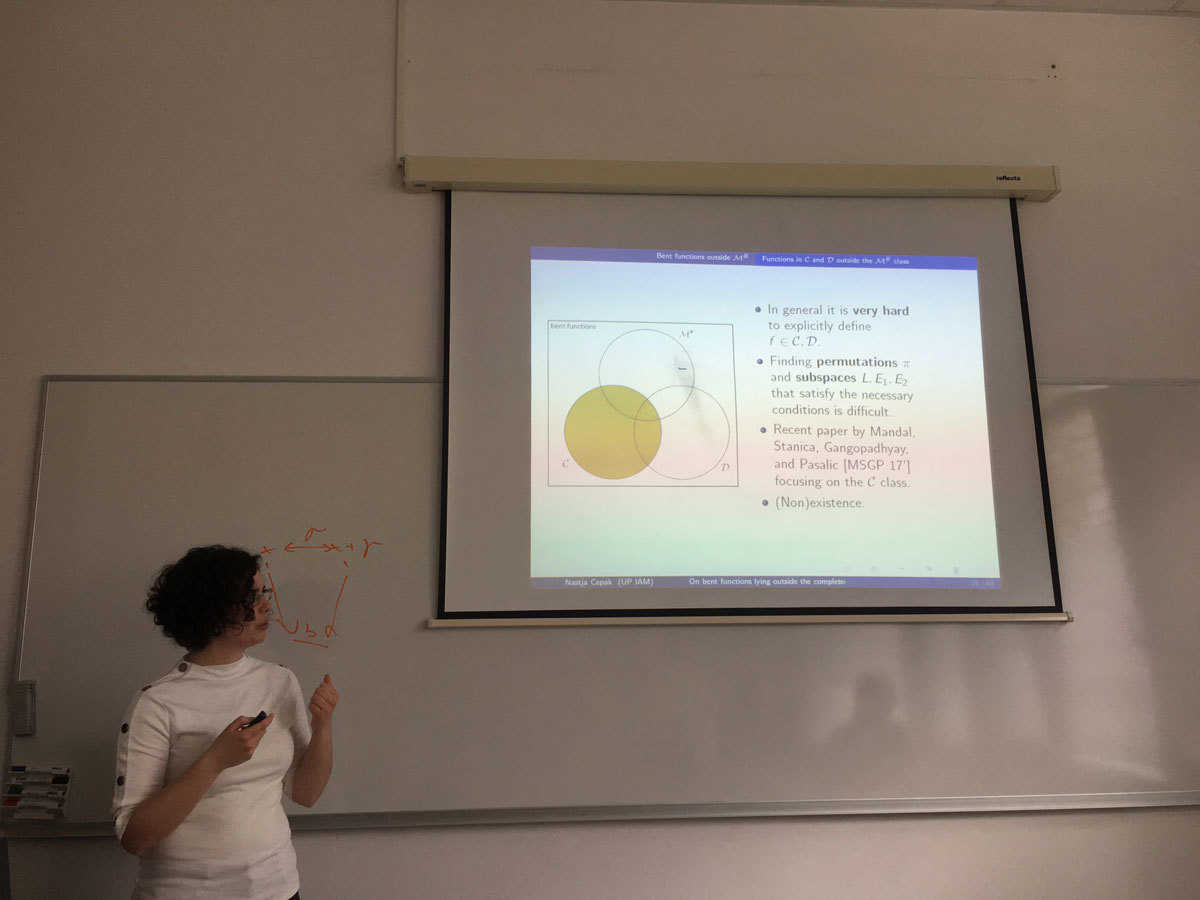Datum in ura / Date and time: 5.3.18
(10:00-11:00)
Predavalnica / Location: FAMNIT-POŠTA
Predavatelj / Lecturer: Klavdija Kutnar (University of Primorska)
Naslov / Title: The beauty of cubic symmetric graphs
Vsebina / Abstract:

In this talk the properties of cubic symmetric graphs will be described.
Special emphases will be given to a recent characterization of these graphs via the so-called rigid cells.